# VLOOKUP – Retrieve Data from Another Workbook

VLOOKUP is one of the key functions among all lookup & reference functions in Excel. It can scan and retrieve data from a static or dynamic table based on your lookup value. It can perform approximate match or exact match by setting lookup matching modes.

Today, we will show you the way to apply VLOOKUP to retrieve data from lookup table in another workbook (an external workbook). It is similar to applying VLOOKUP to retrieve data in the same workbook or worksheet. In our daily work, we often need to extract data from an external workbook, if this is a confusing question for you, today’s article will help you to solve your problem.

## EXAMPLE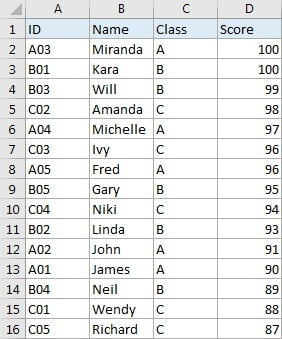Table1: This table is displayed in “workbook1->sheet1”.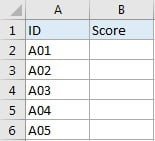Table2: This table is displayed in “workbook2->sheet1”.

In this example, we want to get score from “Score” column of table1 in workbook1->sheet1 to fill “Score” column of table2 in workbook2->sheet1 correspondingly.

We can see that table1 is a summary and all IDs from table2 can be found in this table. Besides, they both list ID as the first column. So, even though they are saved in different workbooks, we still can use VLOOKUP function to perform an exact match lookup to get score for each student.

## ANALYSIS

This is a typical example that can be solved by VLOOKUP function. Different from common examples of VLOOKUP function, in this instance, there is no score information saved in table2, only IDs are sorted in the ID column. And scores are only sorted in the last column of table1 in an external workbook, this table is our lookup table. To retrieve data from lookup table in another workbook, we need to add [Workbook1.xlsx]Sheet1! before range reference.

Actually, when entering VLOOKUP the second argument “table_array”, you can directly move to the workbook->worksheet you can find your lookup table (then this worksheet is displayed on top), drag and drop the lookup table, then [Workbook1.xlsx]Sheet1! with range reference is entered automatically and shown in the formula bar properly.

We create below formula contains VLOOKUP for this instance:

=VLOOKUP(A2,[Workbook1.xlsx]Sheet1!\$A\$2:\$D\$16,4,FALSE)

//You can replace “Workbook1”, ”Sheet1” with your workbook and sheet names.

## FORMULA

Input formula =VLOOKUP(A2,[Workbook1.xlsx]Sheet1!\$A\$2:\$D\$16,4,FALSE) into B2, press ENTER, verify that score “90” is returned by formula for ID ‘A01” properly. You can directly drag the handle down to copy down the formula.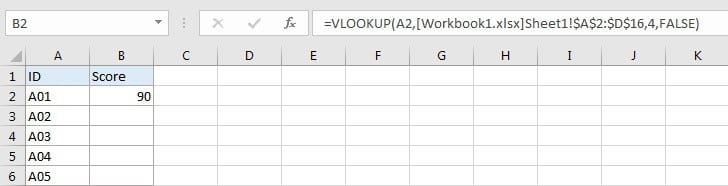## FUNCTION INTRODUCTION

a. VLOOKUP function looks up the input value from the first column of the given table, and returns the value we want from a particular position (row number depends on the row of lookup value, column number depends on the input argument col_index_num).

Syntax:

`=VLOOKUP(lookup_value, table_array, col_index_num,[range_lookup])`

For argument “range_lookup”, there are two matching modes “True” and “False”.

• The default mode is “True”, so if this optional argument is omitted, this value is set to “True” automatically.
• If we set “range_lookup” to “True”, VLOOKUP function performs an approximate match.
• If we set “range_lookup” to “False”, VLOOKUP function looks up data restrictively by performing an exact match. If look up value is not found in table, formula returns error “#N/A”.

## EXPLANATION

`=VLOOKUP(A2,'All Classes'!\$A\$2:\$D\$16,4,FALSE)`

lookup_value is input into A2 (ID “A01”)

table_array is [Workbook1.xlsx]Sheet1!\$A\$2:\$D\$16

col_index_num is 4. Because scores are saved in the fourth column of this table.

range_lookup is set to FALSE. VLOOKUP function performs an EXACT match.

a. In this formula, the look up value is “A01”, VLOOKUP scans this value from the first column of table in workbook1->worksheet1. It continues scanning until it meets the value that is exactly the same with lookup value. In this case, VLOOKUP function will stop scanning when meeting “A01” and stay in current row.

b. Table array is [Workbook1.xlsx]Sheet1!\$A\$2:\$D\$16.

//Workbook1 -> the name of the external workbook; name is enclosed in square brackets.

//Sheet1 -> the name of worksheet that containing the lookup table.

//\$A\$2:\$D\$16 -> lookup table range reference.

This table contains lookup value in its first column and expected value in its last column. We add “\$” before row and column to lock this range, so when copying down the formula, the range doesn’t change. Besides, as we mentioned before, workbook name and worksheet name are added before range reference, so VLOOKUP function can find out lookup table from proper workbook and worksheet.

c. As Score column is the fourth column in lookup table, so we enter 4 as col_index_num. VLOOKUP function retrieves data at the intersection of column “Score” and row “A01”.

d. We set range_lookup mode to “FALSE”, so VLOOKUP function performs an exact match lookup and this operation can ensure that VLOOKUP returns correct score for each student. If lookup ID cannot be found in the given table, an error #N/A will be returned.

## COMMENT

a. The precondition to use this formula is that the external workbook is opened. If this workbook is not opened, and you want to enter table array manually, you should type entire “full path of workbook + workbook name + worksheet name” and enclose them into single quote. See example below: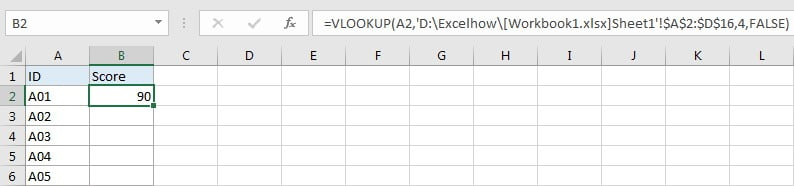b. If workbook name or worksheet contains space in its name, you need to add single quote to enclose the entire [workbook name]sheet name, see example below: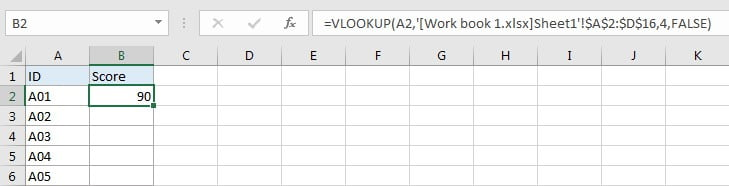### Related Functions

• Excel INDEX function
The Excel INDEX function returns a value from a table based on the index (row number and column number)The INDEX function is a build-in function in Microsoft Excel and it is categorized as a Lookup and Reference Function.The syntax of the INDEX function is as below:= INDEX (array, row_num,[column_num])…
• Excel VLOOKUP function
The Excel VLOOKUP function lookup a value in the first column of the table and return the value in the same row based on index_num position.The syntax of the VLOOKUP function is as below:= VLOOKUP (lookup_value, table_array, column_index_num,[range_lookup])….

Related Posts

VBA Macro For VLOOKUP From Another Sheet

In the previous post, you should know that how to fix or remove the #N/A error when using VLOOKUP formula to lookup value from another sheet. And this post will show you how to use VBA code to vlookup data ...

VLOOKUP From Another Sheet Not Working

In the previous post, you should know that how to fix or remove the #N/A error when using VLOOKUP formula to lookup value from another sheet. And this post will show you reasons why your VLOOKUP formula is not working ...

Fix #N/A Error For VLOOKUP From Another Sheet

This post will show you how to fix the #N/A error why it occurs when you extract values from another sheet using VLOOKUP function in Excel 2016,2013,2010 or other Excel versions. How can you correct a #N/A error in VLOOKUP ...

Get Employee Information by VLOOKUP

VLOOKUP is one of the key functions among all lookup & reference functions in Excel. Today, in this article, we will show you the way to apply VLOOKUP to retrieve employee information. I hope this article will help you in ...

VLOOKUP with Two Lookup Tables

VLOOKUP is one of the key functions among all lookup & reference functions in Excel. Today we will show you the application of VLOOKUP function when there are two lookup tables. EXAMPLE Table1 and table2 record the rates of Y2020 ...

VLOOKUP with Multiple Lookup Values

VLOOKUP is one of the key functions among all lookup & reference functions in Excel. It can scan and retrieve data from a static or dynamic table based on your lookup value. It can perform approximate match or exact match ...

VLOOKUP Data by Date

VLOOKUP is one of the key functions among all lookup & reference functions in Excel. It can scan and retrieve data from a static or dynamic table based on your lookup value. It can perform approximate match or exact match ...

VLOOKUP – Retrieve Data from Another Worksheet

VLOOKUP is one of the key functions among all lookup & reference functions in Excel. It can scan and retrieve data from a static or dynamic table based on your lookup value. It can perform approximate match or exact match ...

Case Sensitive Lookup with SUMPRODUCT and EXACT

Today, we will show you how to use SUMPRODUCT and EXACT to perform a case sensitive exact match. In this article, we provide a simple example to calculate bonus for employees whose names are case-sensitive. If you meet similar scenarios ...

Basic Grade Calculation by VLOOKUP Function – Approximate Match

In Excel, except combination INDEX+MATCH, we can also apply other functions to search data, for example VLOOKUP function. Like MATCH function, VLOOKUP function is one of Excel lookup & reference functions that can perform approximate match or exact match by ...

Sidebar